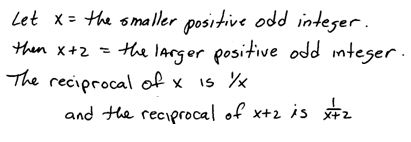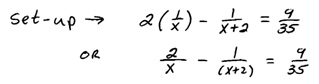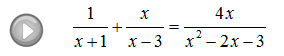## Algebra

### Solving Rational Equations

Rational equations are simply equations with rational expressions in them.

Use the technique outlined earlier to clear the fractions of a rational equation.  After clearing the fractions, we will be left with either a linear or a quadratic equation that can be solved as usual.

Step 1: Factor the denominators.
Step 2: Identify the restrictions.
Step 3: Multiply both sides of the equation by the LCD.
Step 4: Solve as usual.
Step 5: Check answers against the set of restrictions.This process sometimes produces answers that do not solve the original equation (extraneous solutions), so it is extremely important to check your answers.
Tip: It suffices to check that the answers are not restrictions to the domain of the original equation.

Solve.Determining the LCD is often the most difficult part of the process. Use one of each factor found in all denominators.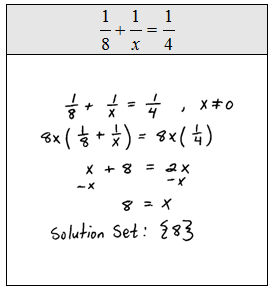Because of the distributive property, multiplying both sides of an equation by the LCD is equivalent to multiplying each term by that LCD as illustrated in the following examples.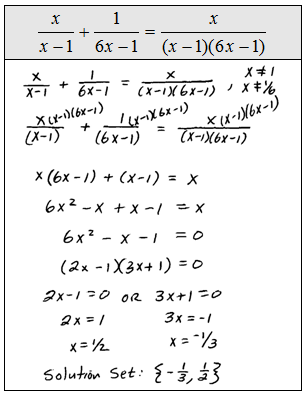Some literal equations, often referred to as formulas, are also rational equations. Use the techniques of this section and clear the fractions before solving for the particular variable.

Solve for the specified variable.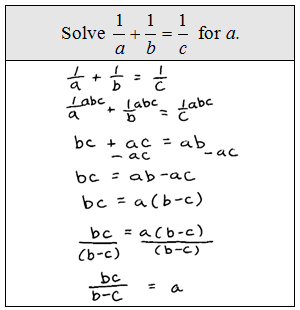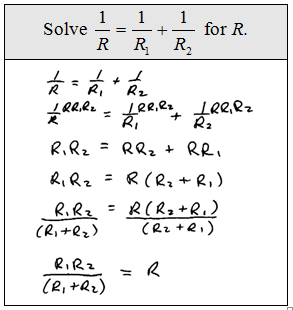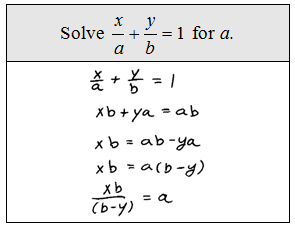The reciprocal of a number is the number we obtain by dividing 1 by that number.

Word Problem: The reciprocal of the larger of two consecutive positive odd integers is subtracted from twice the reciprocal of the smaller and the result is 9/35. Find the two integers.